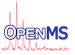OpenMS  2.8.0
AxisTickCalculator Class Reference

Calculates ticks for a given value range. More...

`#include <OpenMS/VISUAL/AxisTickCalculator.h>`

## Public Types

typedef std::vector< std::vector< double > > GridVector
Typedef for the grid vector. More...

## Static Public Member Functions

static void calcGridLines (double x1, double x2, GridVector &grid)
Returns a GridVector with ticks for linear scales. More...

static void calcLogGridLines (double x1, double x2, GridVector &grid)
Returns a GridVector with ticks for logarithmic scales. More...

## Private Member Functions

AxisTickCalculator ()
Constructor: only static methods. More...

## Detailed Description

Calculates ticks for a given value range.

It has only static methods, that's why the constructor is private.

## ◆ GridVector

 typedef std::vector > GridVector

Typedef for the grid vector.

## ◆ AxisTickCalculator()

 AxisTickCalculator ( )
private

Constructor: only static methods.

## ◆ calcGridLines()

 static void calcGridLines ( double x1, double x2, GridVector & grid )
static

Returns a GridVector with ticks for linear scales.

Parameters
 x1 minimum value x2 maximum value grid the grid_vector to fill

## ◆ calcLogGridLines()

 static void calcLogGridLines ( double x1, double x2, GridVector & grid )
static

Returns a GridVector with ticks for logarithmic scales.

Parameters
 x1 minimum value x2 maximum value grid the grid_vector to fill